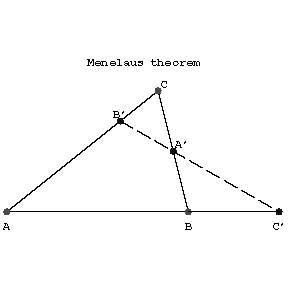# Menelaus theorem

A theorem on the relations between the lengths of the segments on the sides of a triangle determined by an intersecting straight line. It asserts that if the given line intersects the sides of a triangle $ABC$ (or their extensions) at the points $C'$, $A'$ and $B'$, then

$$\frac{AC'}{BC'}\cdot\frac{BA'}{CA'}\cdot\frac{CB'}{AB'}=1.$$

Menelaus' theorem is a particular case of the Carnot theorem; it can be generalized to the case of a polygon. Thus, suppose that a straight line $l$ intersects the edges $A_1A_2,\dots,A_{n-1}A_n,A_nA_1$ of a polygon $A_1\dots A_n$ at the respective points $a_1,\dots,a_n$. Then the following relation is valid:

$$\frac{A_1a_1}{A_2a_1}\cdots\frac{A_{n-1}a_{n-1}}{A_na_{n-1}}\cdot\frac{A_na_n}{A_na_n}=1.$$

The theorem was proved by Menelaus (1st century) and apparently it was known to Euclid (3rd century B.C.).Figure: m063400a